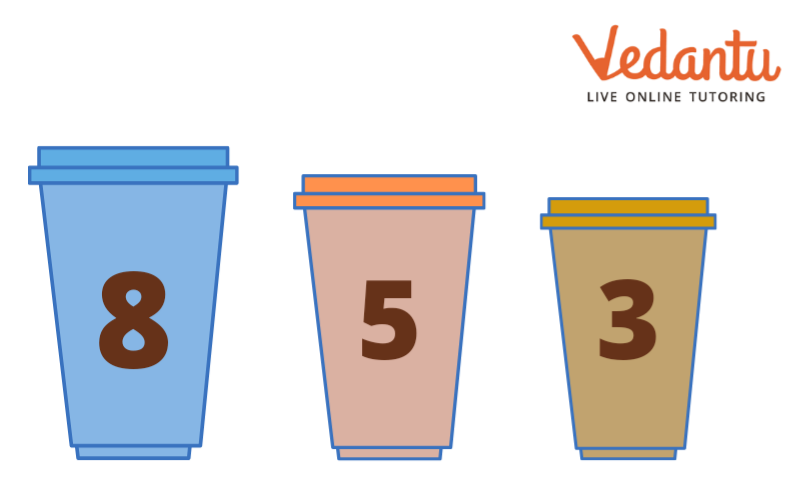Courses
Courses for Kids
Free study material
Free LIVE classes
More

# Learn Maths with Cups - Easy Activity for KidsLIVE
Join Vedantu’s FREE Mastercalss

## What is Cup Maths?

We know that a cup is an item that holds something and that something is a volume. Now, a question arises: what is the use of cups in learning Maths? Well, today we are going to figure this out. Also, you will get to learn basic arithmetic operations on a set of numbers using a cup.

This will be fun for you all as writing numbers on a cup and then performing operations simultaneously will brush your mental calculations and also help you solve a variety of Maths questions that will not only help you at present but also in the future.

Now, let us start with a basic cup Maths example and proceed with certain questions based on it.

## Example of a Cup Maths

Take three disposable cups and start writing numbers on one cup. Suppose you wrote 12 one-digit numbers on one cup, now, take the second cup and use all four Mathematical operators like +, -, and x. These four operators were for the first four numbers. Now, repeat the cycle and in this way, this cycle repeats three times to cover all 12 numbers.

Take the third cup and write numbers as per the numbers you took in the first cup and the operators you used on the second cup.

So, let us take the following numbers in the first cup:

1. 2

2. 5

3. 6

4. 8

5. 5

6. 9

7. 8

8. 6

9. 5

10. 7

11. 4

12. 9Cup Maths

Now, look at the following table and the corresponding operators that we used in the first and the second cup, respectively.

 First Cup Second Cup Third Cup 2 + 5 5 - 3 6 x 6 8 2 5 + 8 9 - 5 8 x 8 6 3 5 + 5 7 - 3 4 x 8 9 3

Here, we are going to take the fourth cup to show the results:

 First Cup Second Cup Third Cup Fourth Cup 2 + 5 7 5 - 3 2 6 x 6 36 8 2 4 5 + 8 13 9 - 5 4 8 x 8 64 6 3 2 5 + 5 10 7 - 3 4 4 x 8 32 9 3 3

We know that the result will be the same as mentioned in the above table. However, we have to sharpen the mental calculation of a kid, so let us change the order of numbers in the fourth cup, as shown below:

 First Cup Second Cup Third Cup Fourth Cup 2 + 3 8 5 - 2 2 6 x 1 3 8 4 6 5 + 3 5 9 - 4 5 8 x 1 3 6 2 3 5 + 1 8 7 - 4 3 4 x 2 5 9 3 5

Now, we will give it to the child so that he/she can arrange the numbers by looking at the first, second, and third cup to give the correct result in the fourth cup.

After he/she is done with the arranging part, check the solution. If he/she gets wrong somewhere, guide him/her by taking images of numbers or objects in surroundings or whatever suits you the best.

Hence, we conclude that cup Maths is an interesting and easy activity for kids to adopt the habit of mental calculation at a very young age. Practising this on a daily basis will master him/her and make a pro in Maths, which, in turn, will be helpful in the long run.

Last updated date: 26th Sep 2023
Total views: 138k
Views today: 3.38k

## FAQs on Learn Maths with Cups - Easy Activity for Kids

1. What are the basic operations in Mathematics? Give examples.

Basic arithmetic operations are Addition, Subtraction, Multiplication and Division and they hold the following significance:

• Addition (Finding the Sum/total; '+')

• Subtraction (Finding the difference; '-')

• Multiplication (Finding the product or multiplication; '×' )

• Division (Finding the quotient; '÷')

• Equal to (=)

2. What is the purpose of arithmetic operators?

An arithmetic operator is a Mathematical concept that takes two operands (values) and performs a calculation on them. They are used in common arithmetic that comprise a set of such operators that can be used within equations to perform a number of types of calculation.